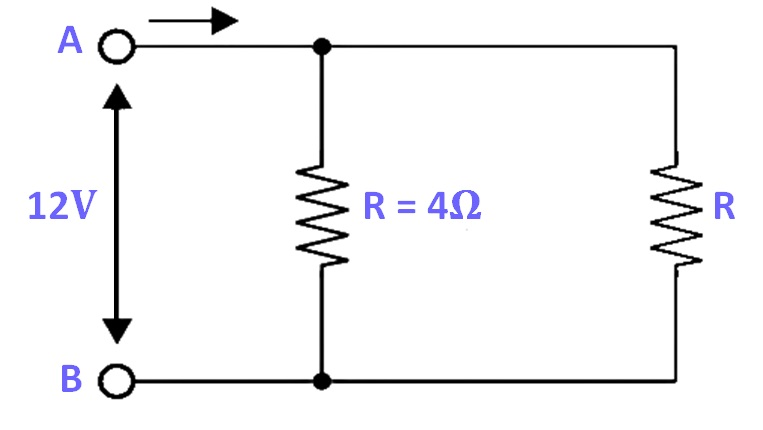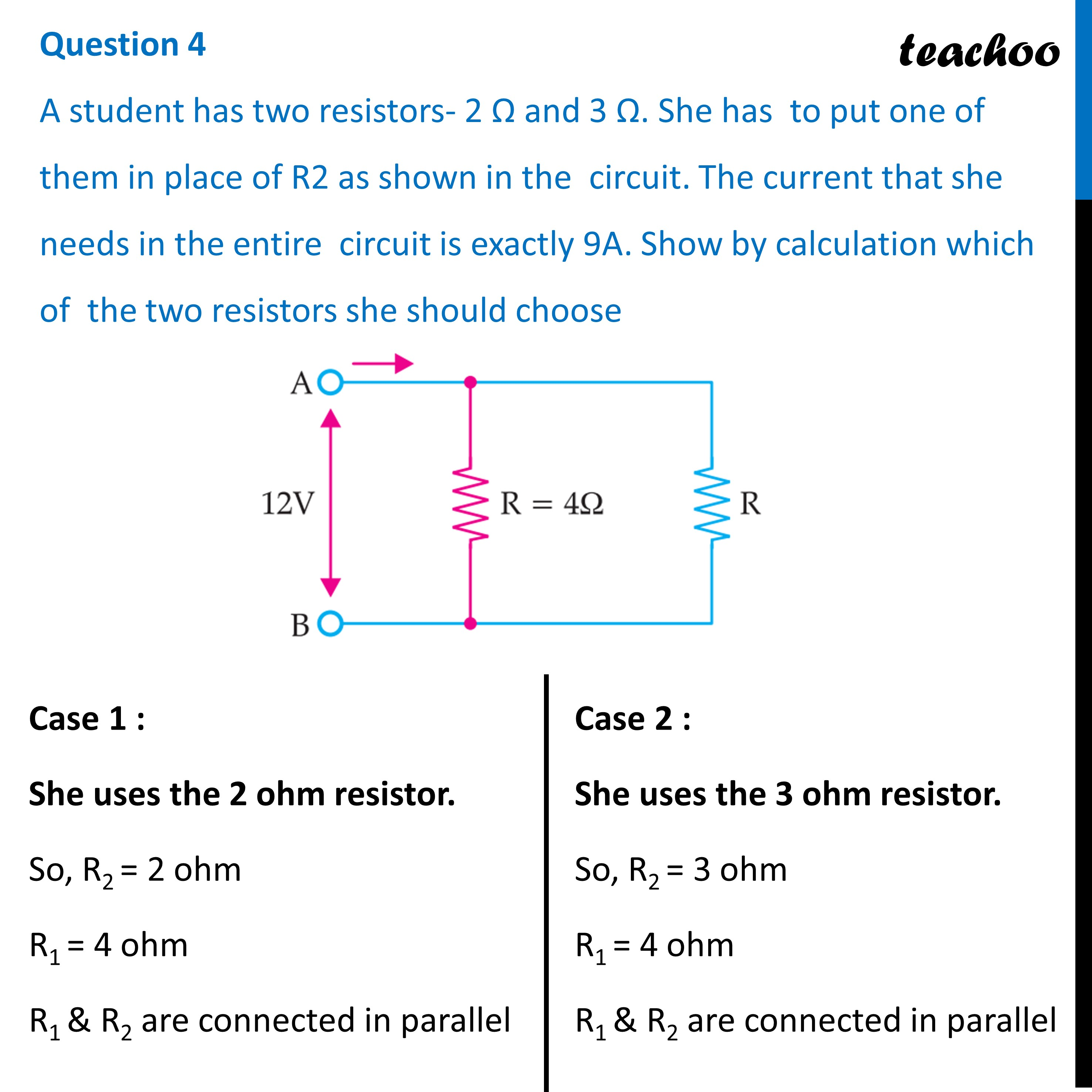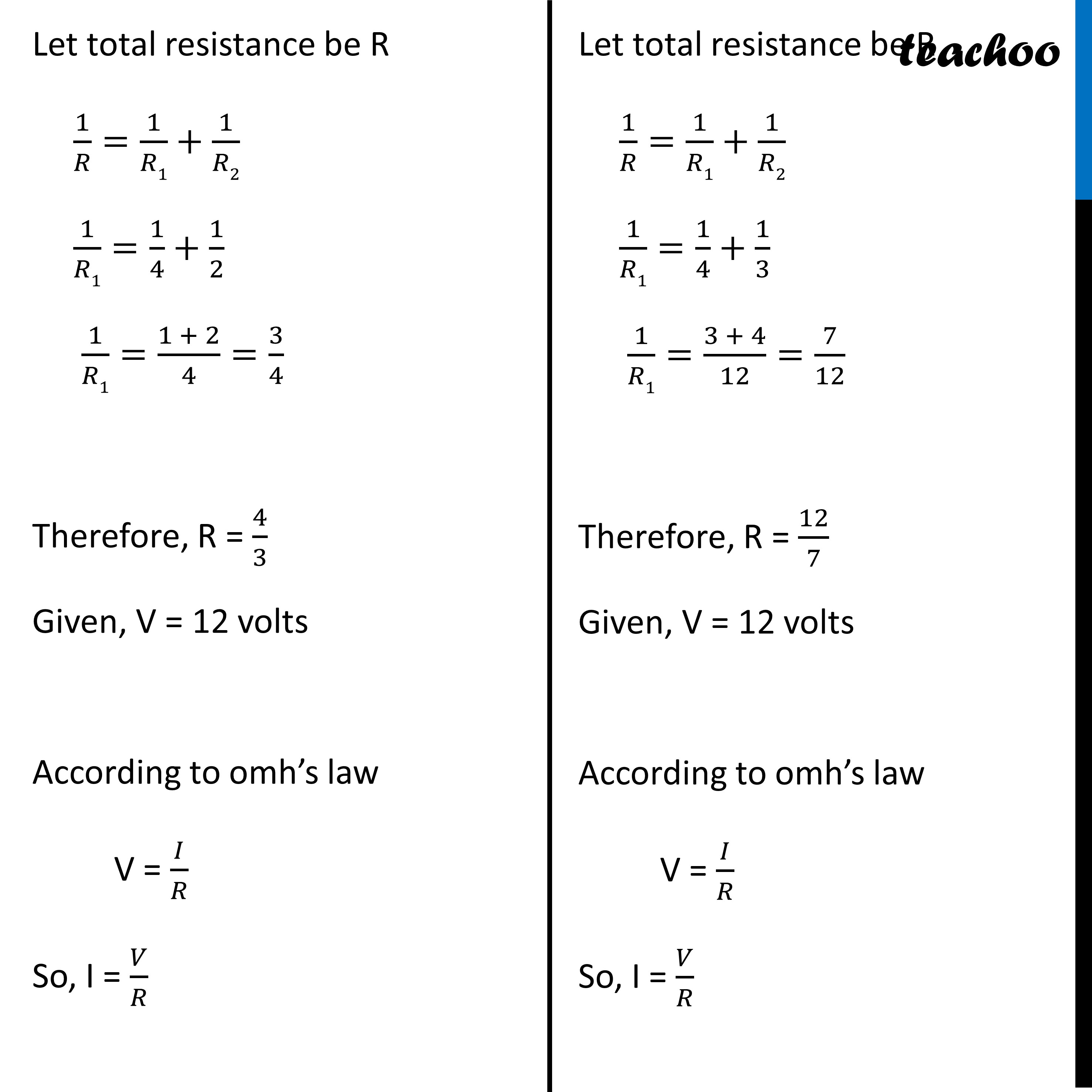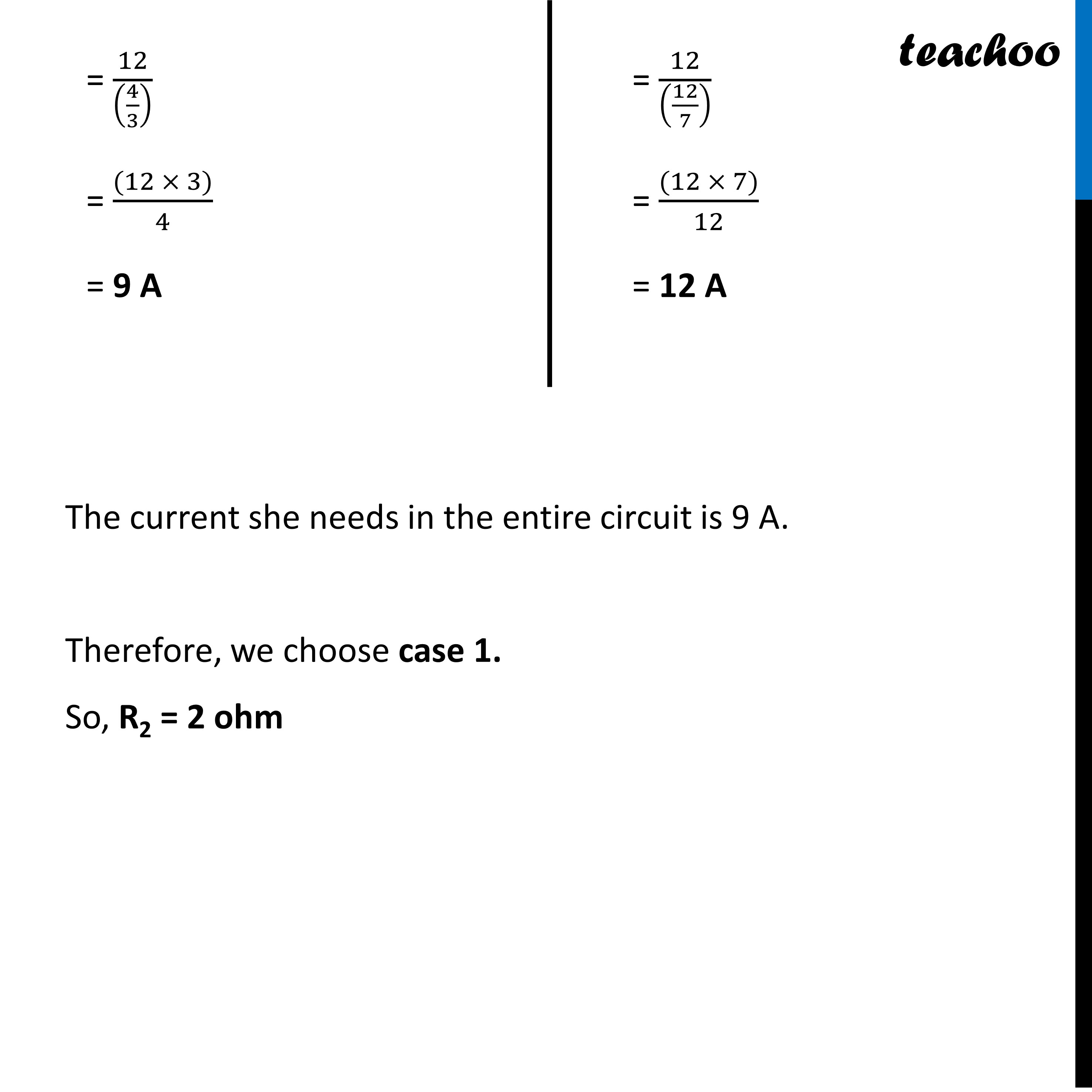Past Year - 2 Mark Questions

Class 10
Chapter 12 Class 10 - Electricity

## A student has two resistors- 2 Ω and 3 Ω. She has to put one of them in place of R2 as shown in the circuit. The current that she needs in the entire circuit is exactly 9A. Show by calculation which of the two resistors she should chooseThe current she needs in the entire circuit is 9 A.

Therefore, we choose case 1.

So, R 2 = 2 ohm

Learn in your speed, with individual attention - Teachoo Maths 1-on-1 Class

### Transcript

Question 4 A student has two resistors- 2 Ω and 3 Ω. She has to put one of them in place of R2 as shown in the circuit. The current that she needs in the entire circuit is exactly 9A. Show by calculation which of the two resistors she should choose Case 1 : She uses the 2 ohm resistor. So, R2 = 2 ohm R1 = 4 ohm R1 & R2 are connected in parallelCase 2 : She uses the 3 ohm resistor. So, R2 = 3 ohm R1 = 4 ohm R1 & R2 are connected in parallel Let total resistance be R 1/𝑅=1/𝑅1+1/𝑅2 1/𝑅1=1/4+1/2 1/𝑅1=(1 + 2)/4=3/4 Therefore, R = 4/3 Given, V = 12 volts According to omh’s law V = 𝐼/𝑅 So, I = 𝑉/𝑅Let total resistance be R 1/𝑅=1/𝑅1+1/𝑅2 1/𝑅1=1/4+1/3 1/𝑅1=(3 + 4)/12=7/12 Therefore, R = 12/7 Given, V = 12 volts According to omh’s law V = 𝐼/𝑅 So, I = 𝑉/𝑅= 12/((4/3) ) = ((12 × 3))/4 = 9 A= 12/((12/7) ) = ((12 × 7))/12 = 12 A The current she needs in the entire circuit is 9 A. Therefore, we choose case 1. So, R2 = 2 ohm